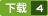### MATLAB Graphics and Data Visualization Cookbook 评分:

1.Collection of data visualization recipes with functionalized versions of common tasks for easy integration into your data analysis workflow 2.Recipes cross-referenced with MATLAB product pages and MATLAB Central File Exchange resources for improved coverage 3.Includes hand created indices to find

...展开详情
2015-03-31 上传 大小：9.61MB

### 评论下载该资源后可以进行评论共1条

2018-01-04MATLAB Graphics and Data Visualization Cookbook 立即下载MATLAB Graphics 立即下载Matlab - Graphics and GUIs with Matlab, 3rd Edition 立即下载Graphics and GUIs with MATLAB 立即下载Graphics and GUIs with matlab 2, third edition 立即下载Graphics and GUIs with matlab 3, third edition 立即下载graphics and guis with matlab 1, third edition 立即下载Matlab - Introducing to Matlab and it's Graphics Capabilities.pdf 立即下载Graphics and GUIs with MATLAB, 3rd Ed (2003)(CRC Press) 立即下载Graphics_and_GUIs_with_MATLAB 立即下载MATLAB® and Design Recipes for Earth Sciences 立即下载MATLAB自学原版教材 A Guide to Matlab For Beginners and Experienced Users 立即下载Essential MATLAB for Engineers and Scientists 6ed 立即下载Using R for Data Analysis and Graphics 立即下载Matlab Primer 立即下载Advanced Mathematics and Mechanics Applications Using MATLAB - Howard B. Wilson with examples 立即下载MATLAB A Practical Introduction to Programming and Problem Solving (4th Edition) 立即下载MATLAB 教程 立即下载html+css+js制作的一个动态的新年贺卡 立即下载qBittorrent插件集合(22个) 立即下载royalosyin